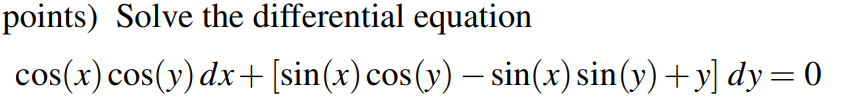Home / Expert Answers / Other Math / points-solve-the-differential-equation-cos-x-cos-y-d-x-sin-x-c-pa367

# (Solved): points) Solve the differential equation $\cos (x) \cos (y) d x+[\sin (x) \c ...??????? points) Solve the differential equation \[ \cos (x) \cos (y) d x+[\sin (x) \cos (y)-\sin (x) \sin (y)+y] d y=0$

We have an Answer from Expert